Ministério da Ciência, Tecnologia, Inovações e Comunicações CBPFIndex CENTRO BRASILEIRO DE PESQUISAS FÍSICAS14/08/2022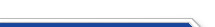Usuário: Senha: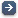Crie sua conta aqui · Busca rápida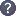Procurar por: Publicações Projetos EventosIr para a busca avançada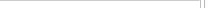Notas de Física
 A three-dimensional superconformal quantum mechanics with $sl(2|1)$ dynamical symmetry Data do cadastro: 21/08/2019 Publicação/Divulgação: 21/08/2019 Resp. pelo cadastro: Status atual: Publicado Ano da publicação: 2019 Descrição: We construct a three-dimensional superconformal quantum mechanics (and its associated de Alfaro-Fubini-Furlan deformed oscillator) possessing an $sl(2|1)$ dynamical symmetry. At a coupling parameter $\beta\neq 0$ the Hamiltonian contains a $\frac{1}{r^2}$ potential and a spin-orbit (hence, a first-order differential operator) interacting term. At $\beta=0$ four copies of undeformed three-dimensional oscillators are recovered. The Hamiltonian gets diagonalized in each sector of total $j$ and orbital $l$ angular momentum (the spin of the system is $\frac{1}{2}$). The Hilbert space of the deformed oscillator is given by a direct sum of $sl(2|1)$ lowest weight representations. The selection of the admissible Hilbert spaces at given values of the coupling constant $\beta$ is discussed. The spectrum of the model is computed. The vacuum energy (as a function of $\beta$) consists of a recursive zigzag pattern. The degeneracy of the energy eigenvalues grows linearly up to $E\sim \beta$ (in proper units) and quadratically for $E>\beta$. The orthonormal energy eigenstates are expressed in terms of the associated Laguerre polynomials and the spin spherical harmonics. The dimensional reduction of the model to $d=2$ produces two copies (for $\beta$ and $-\beta$, respectively) of the two-dimensional $sl(2|1)$ deformed oscillator. The dimensional reduction to $d=1$ produces the one-dimensional $D(2,1;\alpha)$ deformed oscillator, with $\alpha$ determined by $\beta$. Número: CBPF-NF-002/19 Autores: F. Toppan; Ivan E. Cunha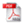Download do PDF (1.027 KB)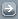Todos EventosTodos PublicaçõesTodasArtigos de Div. CientíficaArtigos em Revistas Nac.Artigos em Revistas Int.Artigos em Eventos Nac.Artigos em Eventos Int.Livros InteirosCapítulos de LivroPropriedades IntelectuaisÁudioPatentesProgramas de computadorModelos de utilidadeMarcasOutras prop. intel.TecnologiasKnow-howServiços tecnológicosTecnologias inovadorasTeses de DoutoradoDissertações de MestradoProjetos de GraduaçãoCiência e SociedadeDocumentos HistóricosMonografiasNotas de FísicaNotas TécnicasOutras Produções
 · Principal    |    CBPF    |    · Copyright 2004 - CBPF. Rio de Janeiro/BrasilCentro Brasileiro de Pesquisas Físicasv 1.1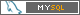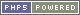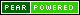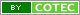Pï¿½g. gerada em 0.01 s Total de consultas SQL: 19